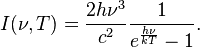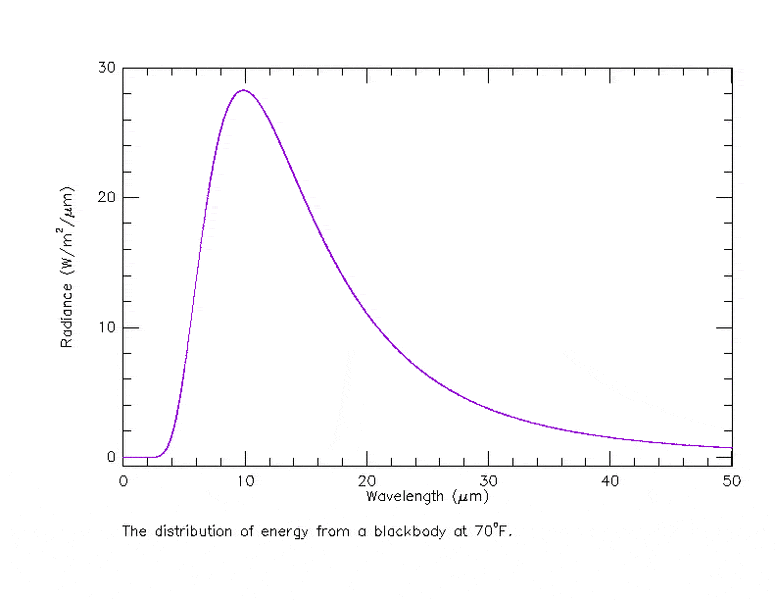# Black Body Radiation - graphical trend

chris_0101

## Homework Statement

To make a long question short, we have the Planck distribution function for a blackbody and the question basically asks to sketch the behavior of this function and explain how one figured these trends.

## Homework Equations

Planck Distribution formula:## The Attempt at a Solution

I am pretty much certain that the Planck Distribution function looks like this:If we were to observe the formula separately, we can see one part of the function is an inverse, thus will decrease, which is shown on the graph. But this is just an observation, and simply does not make that much sense. If anyone can point me into the right direction on how to explain this trend, it would greatly be appreciated.

Thanks